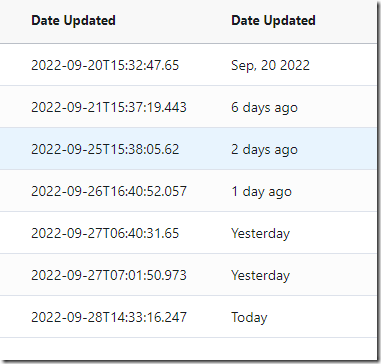# TypeScript: Creating a date filter to show ‘days ago’ in ag-grid

Though I’ve wrote this function for Angular – ag-grid, this can be used as an independent method as well.Code:

`` LastUpdated(value: string):string {   let inputDate = new Date(value);   let today = new Date();   let yesterday = new Date(today.setDate(today.getDate()-1));   let numdays = Math.round((today.getTime() - inputDate.getTime()) / (1000*3600*24));   let ret:string = formatDate(inputDate,"MMM, dd yyyy",'en-US', '+4');   if ( inputDate.setHours(0,0,0,0) == new Date().setHours(0,0,0,0)) {     ret = "Today";   } else if ( inputDate.setHours(0,0,0,0) ==  (yesterday.setHours(0,0,0,0))) {     ret = "Yesterday";   } else if (numdays >= 1 && numdays < 7) {     ret = numdays.toString() + " day" + ((numdays > 1)?'s':'') + " ago";   }   return ret; } ``